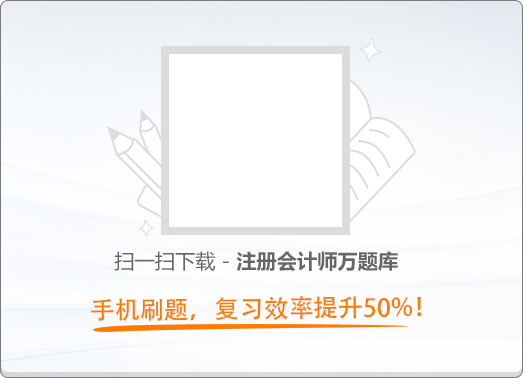150:002019年注册会计师《公司战略与风险管理》真题（二）（非完整版）

1
(单项选择题)

• A.

企业对联盟的控制力较强

• B.

更具有战略联盟的本质特征

• C.

有利于企业长久合作

• D.

有利于扩大企业资金实力

• A
• B
• C
• D

2
(单项选择题)

• A.

共同价值观

• B.

相互适应，自行调整

• C.

工作成果标准化

• D.

技艺（知识）标准化

• A
• B
• C
• D

3
(单项选择题)

• A.

运营风险

• B.

法律风险

• C.

财务风险

• D.

社会文化风险

• A
• B
• C
• D

4
(单项选择题)

• A.

风险资本

• B.

损失融资

• C.

保险

• D.

专业自保

• A
• B
• C
• D

5
(单项选择题)

2012年政府颁布了《生活饮用水卫生标准》。然而，由于相关设施的技术等方面的原因，国内一些地区的自来水水质短期内还不能达到标准。同时，近年随着国内经济迅速发展，国民追求健康和高品质生活的愿望不断增高。通过对上述情况的分析，华道公司于2013年从国外引进自来水滤水壶项目，获得成功。本案例中，华道公司外部环境分析所采用的主要方法是（　）。

• A.

产品生命周期分析

• B.

五种竞争力分析

• C.

PEST分析

• D.

钻石模型分析

• A
• B
• C
• D

6
(单项选择题)

2015年，国内研发和制造铁路设备的东盛公司开启了国际化经营战略，在国外成立了多家子公司。东盛公司在国内的母公司保留技术和产品开发的职能，在国外的子公司只生产由母公司开发的产品。东盛公司采取的国际化经营战略类型的特点是（　）。

• A.

全球协作程度低，本土独立性和适应能力高

• B.

全球协作程度高，本土独立性和适应能力低

• C.

全球协作程度高，本土独立性和适应能力高

• D.

全球协作程度低，本土独立性和适应能力低

• A
• B
• C
• D

7
(单项选择题)

2016年，多年成功经营的啤酒生产企业宝泉公司投资新建了一家果蔬饮料生产企业，但因管理不善出现持续亏损。最近宝泉公司组织果蔬饮料生产企业的管理人员到本公司的啤酒生产企业调研、学习，收效良好。宝泉公司所实施的基准分析的类型属于（　）。

• A.

顾客基准

• B.

一般基准

• C.

内部基准

• D.

竞争性基准

• A
• B
• C
• D

8
(单项选择题)

• A.

经营哲学的变化

• B.

宗旨的变化

• C.

公司目的的变化

• D.

战略层次的变化

• A
• B
• C
• D

9
(单项选择题)

• A.

内幕交易

• B.

掠夺性融资

• C.

直接占用资源

• D.

超额股利

• A
• B
• C
• D

10
(单项选择题)

• A.

马尔科夫分析法

• B.

失效模式影响和危害度分析法

• C.

情景分析法

• D.

敏感性分析法

• A
• B
• C
• D

11
(单项选择题)

• A.

高经营风险与低财务风险

• B.

高经营风险与高财务风险

• C.

低经营风险与高财务风险

• D.

低经营风险与低财务风险

• A
• B
• C
• D

12
(单项选择题)

• A.

概率值

• B.

最大可能损失

• C.

期望值

• D.

在险值

• A
• B
• C
• D